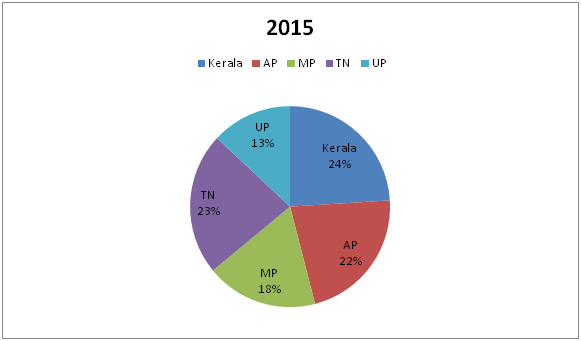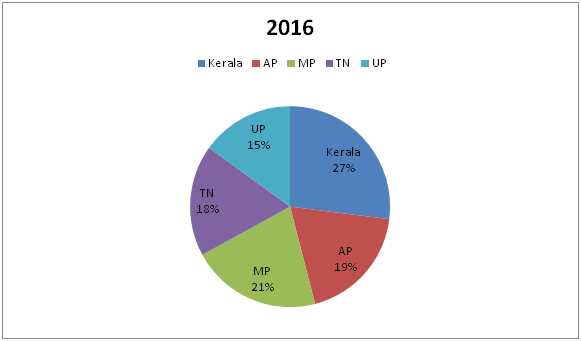# IBPS PO Mains 2018 – Quantitative Aptitude Questions Day-26

Dear Readers, Bank Exam Race for the Year 2018 is already started, To enrich your preparation here we have providing new series of Practice Questions on Quantitative Aptitude – Section. Candidates those who are preparing for IBPS PO Mains 2018 Exams can practice these questions daily and make your preparation effective.

[WpProQuiz 4267]

Directions (Q. 1 – 5) Study the following information carefully and answer the given questions:

The following pie chart 1 and 2 shows the percentage distribution of total number of readers of a magazine in the year 2015 and 2016 among different states.1) If the total number of readers of the state AP in the year 2015 and 2016 is 57200 and 45600 respectively, then find the difference between the total number of readers of the state Kerala in the year 2015 to that of 2016?

a) 3200

b) 2700

c) 2400

d) 3500

e) None of these

2) If the ratio of total number of readers of the state AP in the year 2016 to that of the state MP in the year 2015 is 4 : 5, then find the ratio of total number of readers of all the given states together in the year 2016 to that in the year 2015?

a) 72 : 95

b) 67 : 88

c) 53 : 92

d) 61 : 76

e) None of these

3) If the total number of readers of all the given states together in the year 2015 and 2016 is 24 lakhs and 28 lakhs respectively, then find the total number of readers of the state MP in the year 2015 and 2016 together?

a) 11.5 lakhs

b) 14.6 lakhs

c) 17.3 lakhs

d) 10.2 lakhs

e) None of these

4) Total number of readers of state AP in the year 2016 is approximately what percentage of total number of readers of state MP in the year 2015? (Assume that the total number of readers for both years is same.)

a) 94 %

b) 106 %

c) 88 %

d) 72 %

e) 118 %

5) If total number of readers of state Kerala in the year 2015 is 84000 and the total number of readers of state MP in the year 2016 is 52500, then the ratio of total number of readers of state AP in the year 2015 to that of total number of readers of state Kerala in the year 2016?

a) 157 : 143

b) 181 : 158

c) 165 : 134

d) 154: 135

e) None of these

Directions (Q. 6 – 10): In each of these questions two equations (I) and (II) are given. You have to solve both the equations and give answer as,

a) If x > y

b) If x ≥ y

c) If x < y

d) If x ≤ y

e) If x = y or no relation can be established between x and y

6) I) 3x – 5y = 61

II) 2x – 3y = 38

7) I) x3 × 19 = x2 × 361

II) y1/3 × 17 = 357 ÷ y2/3

8) I) 4x2 – 3 = √2x

II) 5y2 = √5y + 2

9) I) √(x + 4) = √676 – √361

II) y3 – 32568 = 26751

10) I) 4x2 + 20x + 24 = 0

II) 16y2 + 67y + 12 = 0

Direction (1-5) :

The total number of readers of the state AP in the year 2015 = 57200

(22/100)*total number of readers in the year 2015 = 57200

Total number of readers in the year 2015 = 57200*(100/22) = 260000

The total number of readers of the state AP in the year 2016 = 45600

(19/100)*total number of readers in the year 2016 = 45600

Total number of readers in the year 2016 = 45600*(100/19) = 240000

Total number of readers of the state Kerala in the year 2015

= > 260000*(24/100) = 62400

Total number of readers of the state Kerala in the year 2016

= > 240000*(27/100) = 64800

Required difference = 64800 – 62400 = 2400

The ratio of total number of readers of the state AP in the year 2016 to that of the state MP in the year 2015 = 4 : 5(4x, 5x)

Total number of readers in the year 2016 = 4x*(100/19) = 400x/19

Total number of readers in the year 2015 = 5x*(100/18) = 250x/9

Required ratio = (400x/19) : (250x/9) = 72 : 95

The total number of readers of all the given states together in the year 2015 = 24 lakhs

The total number of readers of MP in the year 2015 = 24*(18/100) = 4.32 lakhs

The total number of readers of all the given states together in the year 2016 = 28 lakhs

The total number of readers of MP in the year 2016 = 28*(21/100) = 5.88 lakhs

Required sum = 4.32 + 5.88 = 10.2 lakhs

Required % = (19/18)*100 = 105.55 % = 106 %

Total number of readers of state Kerala in the year 2015 = 84000

Total number of readers of state AP in the year 2015

= > 84000*(100/24)*(22/100)

= > 77000

Total number of readers of state MP in the year 2016 = 52500

Total number of readers of state Kerala in the year 2016

= > 52500*(100/21)*(27/100)

= > 67500

Required ratio = 77000 : 67500 = 154 : 135

Direction (6-10) :

3x – 5y = 61 –à (1)

2x – 3y = 38 –à (2)

By solving the equation (1) and (2), we get,

X = 7, y = -8

X > y

I) x3 × 19 = x2 × 361

X3 ÷ x2 = 361/19

x = 19

II) y1/3 × 17 = 357 ÷ y2/3

Y1/3 × y2/3 = 357/17

Y3/3 = 21

y = 21

x < y

I) 4x2 – √2x – 3 = 0

4x2 – 3√2x + 2√2x – 3 = 0

√2x (2√2x – 3) + 1(2√2x – 3) = 0

(√2x + 1) (2√2x – 3) = 0

x = -1/√2, 3/(2√2) = -0.707, 1.06

II) 5y2 – √5y – 2 = 0

5y2 – 2√5y + √5y – 2 = 0

√5y (√5y – 2) + 1(√5y – 2) = 0

(√5y + 1) (√5y – 2) = 0

y = -1/√5, 2/√5 = -0.448, 0.89

Can’t be determined

I) √(x + 4) = √676 – √361

√(x + 4) = 26 – 19

(x + 4) = 72

X = 49 – 4 = 45

II) y3 – 32568 = 26751

Y3 = 26751 + 32568

Y3 = 59319

Y = ∛59319 = 39

X > y

I) 4x2+ 20x + 24 = 0

4x2 + 8x + 12x + 24 = 0

4x(x + 2) + 12(x + 2) = 0

(4x + 12) (x + 2) = 0

X = -3, -2

II) 16y2 + 67y + 12 = 0

16y2 + 64y + 3y + 12 = 0

16y (y + 4) + 3 (y + 4) = 0

(16y + 3) (y + 4) = 0

Y = -3/16, -4

Can’t be determined

Daily Practice Test Schedule | Good Luck

 Topic Daily Publishing Time Daily News Papers & Editorials 8.00 AM Current Affairs Quiz 9.00 AM Current Affairs Quiz (Hindi) 9.30 AM IBPS Clerk Prelims – Reasoning 10.00 AM IBPS Clerk Prelims – Reasoning (Hindi) 10.30 AM IBPS Clerk Prelims – Quantitative Aptitude 11.00 AM IBPS Clerk Prelims – Quantitative Aptitude (Hindi) 11.30 AM Vocabulary (Based on The Hindu) 12.00 PM IBPS Clerk Prelims – English Language 1.00 PM SSC Practice Questions (Reasoning/Quantitative aptitude) 2.00 PM IBPS PO/Clerk – GK Questions 3.00 PM SSC Practice Questions (English/General Knowledge) 4.00 PM Daily Current Affairs Updates 5.00 PM IBPS PO Mains – Reasoning 6.00 PM IBPS PO Mains – Quantitative Aptitude 7.00 PM IBPS PO Mains – English Language 8.00 PM